Next: Limitations Up: tr01cb1 Previous: Contrasts

# Estimation of Variance Components

In the previous sections it was assumed that all variance terms are known a-priori. In practice, these quantities are unknown and will need to be estimated as part of the model fitting. Variance component estimation is a challenging task in itself, having generated a variety of approaches. Any approach to variance estimation (or combination of approaches) can easily be combined with the multi-level GLM to provide a practical multi-level method; this section discusses some of the more popular approaches.

There are no differences between the first level and any other level from the modelling perspective; however, in practice, estimating the variances is substantially different. At the first-level there typically exists considerable serial auto-correlation (in FMRI time series data) but with a large number of observations. A considerable amount of literature is devoted to specifying the form for the first-level covariance matrix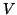and estimating its parameters in the single session case [17,14,19]. In contrast, higher level variance component estimation is typically troubled by having very few observations, while serial auto-correlation between these normally is, and often can be, ignored.

When the number of observations is very low, this imposes restrictions in the types of model which are practically estimable. For instance, while it is possible to formulate a model where the variance about the group mean is different for each session/subject, such a model is not estimable because there is only a single measurement per session/subject.

Several approaches to estimation within the multi-level GLM currently exist, of which the parametric techniques can, most easily, be split into Bayesian and non-Bayesian approaches.

Classically, variance components tend to be estimated separately using iterative estimation schemes employing Ordinary Least Squares (OLS), Expectation Maximisation (EM) or Restricted Maximum Likelihood (ReML), see  for details.

The specific choice of the variance component estimator will also determine the 'effective' degrees of freedom of the variance estimate. For ordinary least squares estimates, the degrees of freedom simply is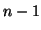. When more sophisticated estimation techniques and/or variance structures are being used, this changes significantly [19,10]; e.g. when enforcing mixed- effects variance to be greater than the fixed-effects variance, this can increase the effective degrees of freedom, a quantity typically estimated using a Satterthwaite approximation .

As an example of a non-Bayesian approach,  estimates variance components at each level of the split-level model separately. First-level estimation incorporates autoregressive AR(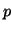) noise estimated from the lag-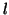-autocovariance matrices and utilises the pre-whitening approach to generate BLUEs for the first-level parameters. At higher levels, they propose EM for estimation of the random effects variance contribution, in order to reduce bias in the variance estimation - a potential problem in higher-level analyses if simple OLS were used (note that  shows that this is not necessary at first-level). Positivity of the random- effects variance, avoiding what is known as the 'negative variance problem'  (where mixed-effects variance estimates are lower than fixed-effects variances implying negative random-effects variance), is partially addressed but not strictly enforced. As a separate stage, in order to boost effective degrees of freedom for later mixed-effects inference, the authors propose to post-hoc spatially regularise the random-effects variance via smoothing a random variance ratio image. As a consequence, the resulting analysis does not produce a mixed-effects analysis. It should be noted that this specific form of spatial regularisation (or indeed any) is not a necessary ingredient of an EM approach to variance estimation.

In contradistinction  have proposed an empirical-Bayesian approach  for estimation of the full single-level model. Unlike the previous case and the techniques advocated in this paper, this relates the parameters of interest to the full set of original data, i.e. does not utilise the 'summary statistics' approach. Parameter and variance component estimation is no longer separated. Conditional posterior point estimates are generated using EM which give rise to posterior probability maps.

More recently,  have placed the higher-level GLM estimation in a fully Bayesian framework. Using appropriate reference priors, the method is based on Markov-Chain-Monte-Carlo sampling from the full posterior distribution of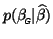. Under the parametric model assumptions, the posterior has a non-central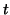-distribution which the method fits to the MCMC samples. This is done in order to both estimate the appropriate degrees of freedom for the mixed-effects inference, and in order to avoid having to sample densely far into the tail of the posterior. As in the empirical-Bayesian case, parameters and their relevant variance components are estimated together. Also, as the number of degrees of freedom is estimated as part of the-distribution fit there is no need to separately approximate this quantity e.g. via the Satterthwaite approximation . This technique provides for an unbiased and efficient estimation of multi- level GLMs within the 'summary statistics' approach, also strictly enforcing positivity of variance components at all levels. This technique, combined with a pre-whitening approach to first-level estimation  has been implemented as part of FSL [1,7].Next: Limitations Up: tr01cb1 Previous: Contrasts
Christian Beckmann 2003-07-16